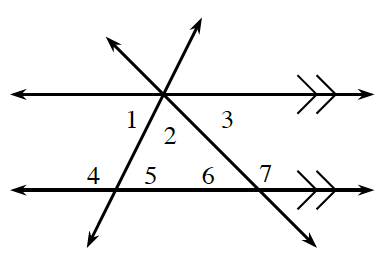### Home > CC3 > Chapter Ch9 > Lesson 9.1.1 > Problem9-9

9-9.

If $m\angle5 = 53º$ and  $m\angle7 = 125º$, find the measures of each numbered angle.  Then explain how you found each angle, citing definitions and conjectures that support your steps.

Since two of the lines are parallel, their alternate interior angles and corresponding angles are equal to each other.

Angles 1, 2, and 3 form a straight angle, as do angles 4 and 5 and angles 6 and 7.

$m∠7 = m∠1 + m∠2$